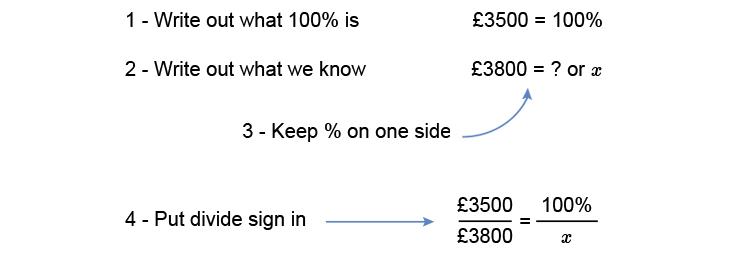# % rate of interest

Example 1

Harriet had £3500 in her savings account. After a year she had £3800. What % rate of interest did she have on her account?Multiply both sides by x to get x on its own (whatever you do to one side you do to the other).

(3500timesx)/3800=(100timescancelx)/cancelx

Multiply both sides by 3800 to get x on its own

(3500xtimescancel3800)/cancel3800=100times3800

3500x=100times3800

Divide both sides by 3500 to get x on its own

(cancel3500x)/cancel3500=(100times38cancel00)/(35cancel00)

x=(100times38)/35

x=108.57%

After a year Harriet had 108.57% of her original amount of £3500. Take away the original amount which is represented by 100% to find out the rate of interest.

108.57 - 100 = 8.57% per annum

Answer: Harriet had 8.57% interest rate